# Karnataka Class 10 Maths Important Questions

How does one prepare for the exams? Read up the textbooks, write down the important formulae? Redo the previous year question papers? Well, each and every student may have their own ways of preparing for the exams. Meanwhile, has anyone ever tried solving the important questions from a subject? How can a student access these important questions?

For future reference, the Karnataka 10th board students can have a look at these important questions given below:

### Karnataka Class 10 Maths Important Questions With Answers

Question 1: Let U = {1, 2, 3, 4, 5, 6}, A = {2, 3} and B = {3, 4, 5}.

Find A′, B′, A′ ∩ B′, A ∪ B and hence show that ( A ∪ B )′ = A′∩ B′.​

Solution:

U = {1, 2, 3, 4, 5, 6} , A = {2, 3} and B = {3, 4, 5}

A’ = {1, 4, 5, 6}

B’ = {1, 2, 6}

A′ ∩ B′ = {1, 6}

(A U B) = {2, 3, 4, 5}

(A ∪ B )′ = {1, 6}

A′ ∩ B′ = {1, 6}

(A ∪ B)′ = A′ ∩ B′

Question 2: Prove that 2 + √3 is an irrational number.

Solution:

If (2 + √3) is rational, then,

(2 + √3) = a / b (say) where a and b are integers and b ≠ 0

⇒ √3 = a / b − (2)

⇒ √3 = [a − 2b] / b …(1)

∵ a and b are integers

∴ a – 2b is also an integer.

⇒ (a − 2b) / b is rational.

The L.H.S. of equation (1) is the square root of a prime number.

So, it is irrational and R.H.S. is rational.

It is a contradiction because a rational number and an irrational number can never be equal.

So, our assumption of 2 + √3 being rational is wrong.

Hence, 2 + √3 is an irrational number.

Question 3: Find the sum of all 2 digit natural numbers that are divisible by 5.

Solution:

The two-digit natural numbers divisible by 5 are 10, 20 …… 90, 95

The series forms an arithmetic progression.

The last term is an = a + (n – 1) d

95 = 10 + (n – 1) 5

95 – 10 = (n – 1) 5

85 / 5 = n – 1

17 = n – 1

17 + 1 = 18 = n

n = 18

Sum of n numbers in a series is given by,

Sn = (n / 2) (a + an)

= (18 / 2) (10 + 95)

= 9 * 105

= 945

Question 4: If 2 (nP2) + 50 = 2nP2, then find the value of n.

Solution:

nPr = n! / (n – r)!

2 (nP2) + 50 = 2nP2

2 * (n! / (n – 2)!) + 50 = 2n! / (2n – 2)!

2 * [n (n – 1) (n – 2)! / (n – 2)!] + 50 = 2n (2n – 1) (2n – 2)! / (2n – 2)!

2n (n – 1) + 50 = 2n (2n – 1)

2n2 – 2n + 50 = 4n2 – 2n

2n2 = 50

n2 = 50 / 2

= 25

n = √25

n = 5

Question 5: Rationalise the denominator and simplify [3√2] / [√5 − √2].

Solution:

[3√2] / [√5 − √2]

= {[3√2] / [√5 − √2]} * {[√5 + √2] / [√5 + √2]}

= [3√2 √5 + 3√2 √2] / [(√5)2 – (√2)2]

= [3√10 + 6] / 3

= 3 (√10 + 2) / 3

= √10 + 2

Question 6: A box has 4 red and 3 black marbles. Four marbles are picked up randomly. Find the probability that two marbles are red.

Solution:

There are 7 marbles, out of these 4 marbles can be drawn in 7C4 = 35 ways.

∴ n (S) = 35

Two marbles out of 4 red marbles can be drawn in 4C2 = 6 ways.

The remaining 2 marbles must be black and they can be drawn in 3C2 = 3 ways.

∴ n (A) = 4C2 3C2 = 6 * 3 = 18

P (A) = n (A) / n (S)

= 18 / 35

Question 7: Calculate the standard deviation for the following scores: 5, 6, 7, 8, 9.

Solution:

 x x2 5 25 6 36 7 49 8 64 9 81 ∑x = 35 ∑x2 = 255

Standard deviation = √(∑x2 / N) – (∑x / N)2

= √(255 / 5) – (35 / 5)2

= √51 – 49

= √2

= 1.414

Question 8: Find the radius of a circle whose centre is (– 5, 4) and which passes through the point (– 7, 1).

Solution:

(x1, y1) = (– 5, 4)

(x2, y2) = (– 7, 1)

∴ d = √(x2 − x1)2 + (y2 − y1)2

Radius of the circle = √[−7 − (−5)]2 + (1 − 4) 2

= √(− 7 + 5)2 + (– 3)2

= √(−2)2 + (−3)2

= √4 + 9

r = √13

Question 9: In Δ ABC, DE | |BC, if AD = 2 cm, DB = 5 cm and AE = 4 cm, find AC.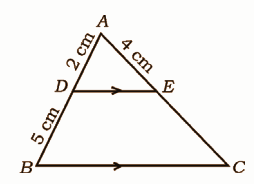Solution:

In Δ ABC, DE || BC

∴ AD / DB = AE / EC [BPT]

2 / 5 = 4 / EC

EC = (4 * 5) / 2

= 10 cm

∴ AC = AE + EC

= 4 + 10

= 14 cm

Question 10: If cos θ = 5 / 13, then find the value of (sin θ + cos θ) / (sin θ – cos θ).

Solution: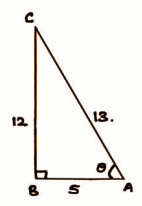cos θ = 5 / 13 = AB / AC

In triangle ABC, B = 90o.

BC2 = AC2 – AB2

BC2 = 132 – 52

BC = √169 – 25

= √144

= 12

sin θ = 12 / 13

(sin θ + cos θ) / (sin θ – cos θ)

= [(12 / 13) + (5 / 13)] / [(12 / 13) – (5 / 13)]

= (17 / 13) * (13 / 7)

= (17 / 7)

Question 11: From the top of a building 20 m high, the angle of elevation of the top of a vertical pole is 30° and the angle of depression of the foot of the same pole is 60°. Find the height of the pole.

Solution: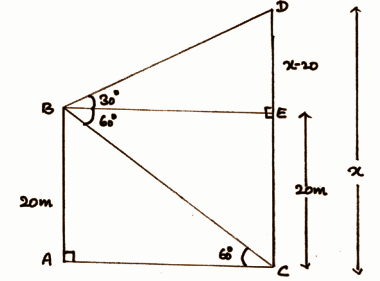In △BED, DBE = 30o

tan 30o = DE / BE

1 / √3 = (x – 20) / BE

BE = √3 (x – 20)

In △ABC, ACB = 60o

tan 60o = AB / AC

√3 = 20 / [√3 (x – 20)]

3 (x – 20) = 20

3x – 60 = 20

3x = 80

x = 80 / 3 = 26.6 cm

Height of the pole = 26.6 cm

Question 12: A solid metallic cylinder of diameter 12 cm and height 15 cm is melted and recast into toys in the shape of a right circular cone mounted on a hemisphere as shown in the figure. If radii of the cone and hemisphere are each equal to 3 cm and the height of the toy is 7 cm, calculate the number of such toys that can be formed.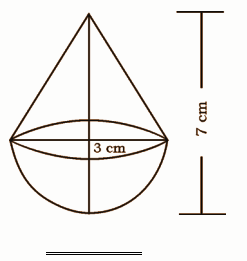Solution:

Height of the cone = h = 24 cm

Slant height of the cone = l = 25 cm.

∴ Lateral Surface Area of the cone = πrl

= π × 7 × 25 sq.cm

= 175 π sq.cm.

Lateral Surface Area of the cylinder = 2πrh

= 2π × 7 × 30 sq.cm

= 420 π sq.cm.

Lateral Surface Area of the hemisphere = 2πr2

= 2π × 72

= 98π sq.cm.

Total Surface Area of the solid = Lateral Surface Area of the cone + Lateral Surface Area of the cylinder + Lateral Surface Area of the hemisphere

= (175 π + 420 π + 98 π) sq.cm.

= (22 / 7) * 693

= 2178 sq.cm.

Cost of painting = (2178 * 10) / 100

= Rs. 217·8

Question 13: Find three consecutive positive integers such that the sum of the square of the first integer and the product of the other two is 92.

Solution:

Let x, (x + 1) and (x + 2) be the three consecutive positive integers.

According to the given,

x2 + (x + 1) (x + 2) = 92

x2 + x2 + 2x + x + 2 = 92

2x2 + 3x + 2 – 92 = 0

2x2 + 3x – 90 = 0

2x2 – 12x + 15x – 90 = 0

2x (x – 6) + 15 (x – 6) = 0

(x – 6) (2x + 15) = 0

x – 6 = 0, 2x + 15 = 0

x = 6, x = – 15 / 2

The value of x cannot be negative.

Therefore, x = 6

Hence, the required three consecutive positive integers are 6, 7 and 8.

Question 14: At constant pressure, a certain quantity of water at 24°C is heated. It was observed that the rise in temperature was found to be 4°C per minute. Calculate the time required to raise the temperature of water to 100°C at sea level by using formula.

Solution:

The temperature of the water is at 24 degrees and raised by 4 degrees per minute. This forms an arithmetic progression 24, 28 , 32, 36, ….100

a = 24

d = 4

Tn = a + (n – 1)d

100 = 24 + (n – 1) 4

76 = (n – 1) 4

76 / 4 = (n – 1)

19 = (n – 1)

19 + 1 = n

20 = n

Question 15: Draw a chord of length 6 cm in a circle of radius 5 cm. Measure and write the distance of the chord from the centre of the circle.

Solution: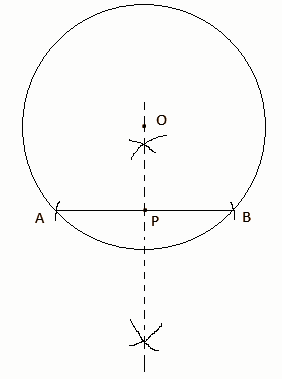Length of chord AB = 6 cm

The distance of the chord from the centre O is OC = 4 cm (by measure)

Question 16: A student while conducting an experiment on Ohm’s law, plotted the graph according to the given data. Find the slope of the line obtained.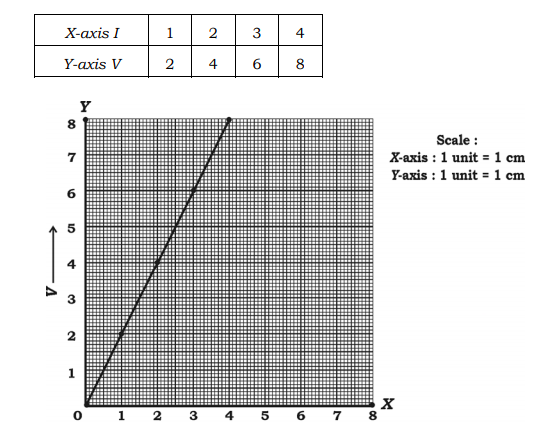Solution:

From the given graph:

(x1, y1) = (1, 2)

(x2, y2) = (2, 4)

(x3, y3) = (3, 6)

(x4, y4) = (4, 8)

Slope = (y4 – y1) / (x4 – x1)

= (8 – 2) / (4 – 1)

= 6 / 3

= 2

Hence, the slope of the line obtained is 2.

Question 17: Draw the plan for the information given below: (Scale 20 m = 1 cm).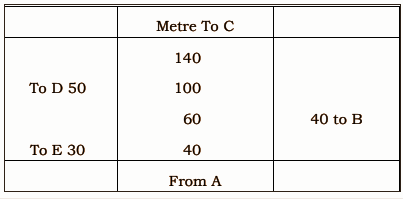Solution: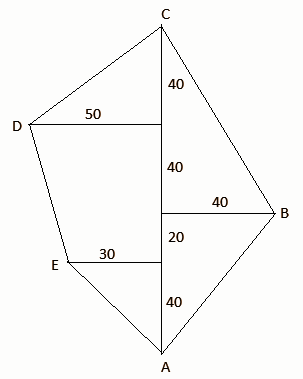Question 18: A dealer sells an article for Rs. 16 and loses as much per cent as the cost price of the article. Find the cost price of the article.

Solution:

Let x be the cost price of the article.

Loss percentage = x%

The selling price of the article = Rs. 16

Loss percentage = (Loss/CP) × 100

x = (loss/x) × 100

x2 = 100 × loss

⇒ Loss = x2 / 100

⇒ x – 16 = x2 / 100

⇒ x2 = 100x – 1600

⇒ x2 – 100x + 1600 = 0

⇒ x2 – 80x – 20x + 1600 = 0

⇒ x (x – 80) – 20 (x – 80) = 0

⇒ (x – 80) (x – 20) = 0

⇒ x = 80, x = 20

Therefore, the cost price of the article is Rs. 20 or Rs. 80.

Question 19: A solid is in the form of a cone mounted on a right circular cylinder, both having the same radii as shown in the figure. The radius of the base and height of the cone is 7 cm and 9 cm respectively. If the total height of the solid is 30 cm, find the volume of the solid.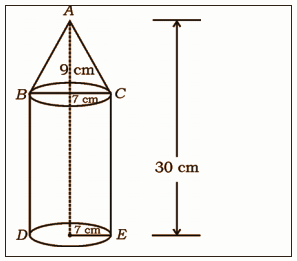Solution:

The radius of the circular base of the cylinder and cone = r = 7 cm

Height of the cone = h = 9 cm

The total height of the solid = 30 cm

Thus, height of the cylinder = H = 30 – 9 = 21 cm

Volume of cone = (1 / 3) πr2h

= (1 / 3) × (22 / 7) × 7 × 7 × 9

= 22 × 7 × 3

= 462 cm3

Volume of cylinder = πr2H

= (22 / 7) × 7 × 7 × 21

= 22 × 7 × 21

= 3234 cm3

Therefore, the total volume of the solid = 462 + 3234 = 3696 cm3.

Question 20: State and prove Basic Proportionality (Thale’s) theorem.

Solution:

Statement: If a line is drawn parallel to one side of a triangle to intersect the other two sides in distinct points, the other two sides are divided in the same ratio.

Proof:

In triangle ABC, a line parallel to side BC intersects other two sides namely AB and AC at D and E respectively.

Join BE and CD.

Also, draw DM ⊥ AC and EN ⊥ AB.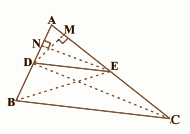Similarly,

Area (∆ BDE) = 1 / 2 (DB × EN)

Area (∆ ADE) = 1 / 2 (AE × DM)

Area (∆ DEC) = 1 / 2 (EC × DM)

Area (∆ ADE) / Area (∆ BDE) = [1 / 2 AD × EN)] / [1 / 2 (DB × EN)]

Area (∆ ADE) / Area (∆ DEC) = [1 / 2 (AE × DM)] / [1 / 2 (EC × DM)]

= AE / EC ….(i)

Triangle BDE and DEC are on the same base DE and between the same parallels.

Therefore, area (∆ BDE) = area (∆ DEC) ….(iii)

From (i), (ii) and (iii),

AD / DB = AE / EC

Hence proved.